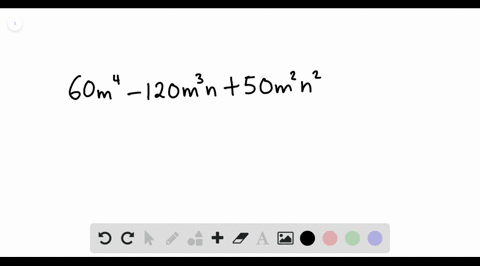Enroll in one of our FREE online STEM summer camps. Space is limited so join now!View Summer Courses### Factor each polynomial. If a polynomial cannot be…

00:55Other Schools

Need more help? Fill out this quick form to get professional live tutoring.

Get live tutoring
Problem 3

Factor each polynomial. If a polynomial cannot be factored, write prime. Factor out the greatest common factor as necessary.
$$13 p^{4} q^{2}-39 p^{3} q+26 p^{2} q^{2}$$

$13 p^{4} q^{2}-39 p^{3} q+26 p^{2} q^{2}=13 p^{2} q\left(p^{2} q-3 p+2 q\right)$

## Discussion

You must be signed in to discuss.

## Video Transcript

where he has to factor this long expression here. Well, look at her coefficients. First we have 13 39 and 26 each week. In fact, her out a factor of 13. Next, we will look at our P ease. So this is Peter the power for future power three and P squared. This means we can factor out P squared and last we look at our accused. We have cues. GERD Q. Another accused grid so it can factor out a cue. So this means we're left with p squared cue from the first term. Negative three p from the second term and positive too cue from the last term.